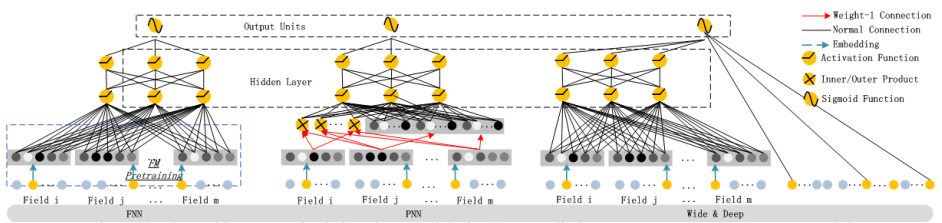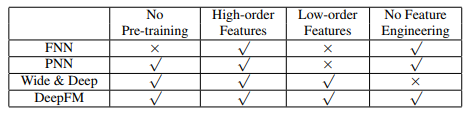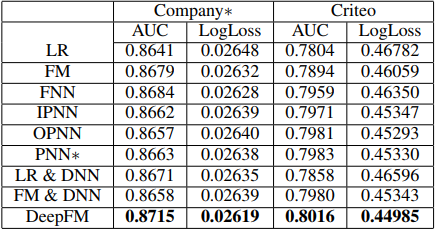# FM系列算法解读（FM+FFM+DeepFM）

## 综述

在计算广告中，CTR是非常重要的一环。对于特征组合来说，业界通用的做法主要有两大类：FM系列和Tree系列。这里我们来介绍一下FM系列。
在传统的线性模型中，每个特征都是独立的，如果需要考虑特征与特征之间的相互作用，可能需要人工对特征进行交叉组合。非线性SVM可以对特征进行核变换，但是在特征高度稀疏的情况下，并不能很好的进行学习。现在有很多分解模型可以学习到特征之间的交互隐藏关系，基本上每个模型都只适用于特定的输入和场景。推荐系统是一个高度系数的数据场景，由此产生了FM系列算法。
本文主要涉及三种FM系列算法：FM，FFM，DeepFM

## 一、FM算法（Factorization Machines）

### 背景

FM(Factorization Machine)主要是为了解决数据稀疏的情况下，特征怎样组合的问题。已一个广告分类的问题为例，根据用户与广告位的一些特征，来预测用户是否会点击广告。数据如下：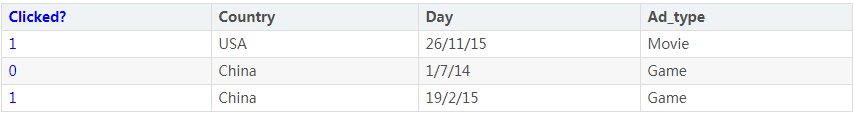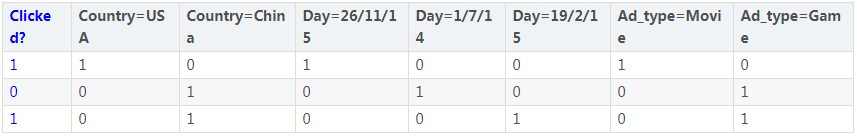one-hot会带来数据的稀疏性，使得特征空间变大。

$y={w}_{0}+\sum _{i=1}^{n}{w}_{i}{x}_{i}+\sum _{i}={1}^{n-1}\sum _{j=i+1}^{n}{w}_{ij}{x}_{i}{x}_{j}$

### FM求解

Wij求解的思路是通过矩阵分解的方法，为了求解Wij，我们队每一个特征分量xi引入辅助向量${V}_{i}=\left({v}_{i1},{v}_{i2},...,{v}_{ik}\right)$$V_i=(v_{i1},v_{i2},...,v_{ik})$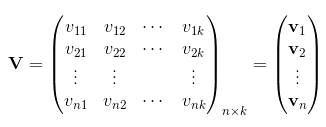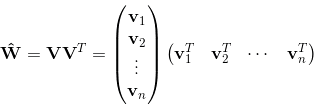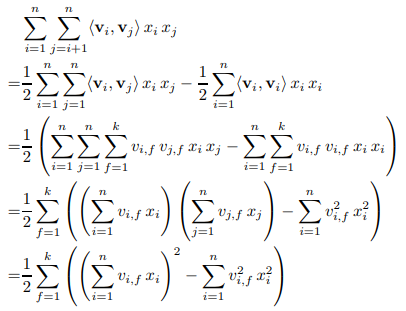FM的复杂度为$O\left(k{n}^{2}\right)$$O(kn^2)$，通过上述等式，FM的二次项化简为只与${v}_{i,f}$$v_{i,f}$有关的等式。因此，FM可以在线性时间对新样本做出预测，复杂度和LR模型一样，且效果提升不少。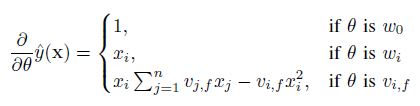$\sum _{j=1}^{n}{v}_{j,f}{x}_{j}$$\sum_{j=1}^nv_{j,f}x_j$只与f有关，只要求出一次所有的f元素，就能够计算出所有${v}_{i,f}$$v_{i,f}$的梯度，而f是矩阵V中的元素，计算复杂度为O(kn)。当已知$\sum _{j=1}^{n}{v}_{j,f}{x}_{j}$$\sum_{j=1}^nv_{j,f}x_j$时计算每个参数梯度的复杂度是O(1)，更新每个参数的复杂度为O(1)，因此训练FM模型的复杂度也是O(kn)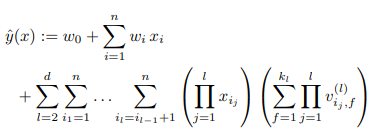### FM vs SVM

SVM和FM的主要区别在于：

• SVM的二元特征交叉参数是独立的，而FM的二元特征交叉参数是两个k维的向量vi、vj，交叉参数就不是独立的，而是相互影响的。
• FM可以在原始形式下进行优化学习，而基于kernel的非线性SVM通常需要在对偶形式下进行
• FM的模型预测是与训练样本独立，而SVM则与部分训练样本有关，即支持向量

## 二、FFM算法（Field-aware Factorization Machine）

$y\left(X\right)={w}_{0}+\sum _{i=1}^{n}{w}_{i}{x}_{i}+\sum _{i=1}^{n}\sum _{j=i+1}^{n}<{V}_{i,{f}_{j}},{V}_{j,{f}_{i}}>{x}_{i}{x}_{j}$

### FFM实现

• 损失函数
FFM将问题定义为分类问题，使用的是logistic loss，同时加入正则项

$mi{n}_{w}\sum _{i=1}^{L}log\left(1+exp\left(-{y}_{i}\varphi \left(w,{x}_{i}\right)\right)\right)+\frac{\lambda }{2}||w|{|}^{2}$

• 梯度下降
梯度下降方法有很多种，根据为提高效率分别衍生了批量梯度下降，随机梯度下降及小批量梯度下降，根据需求选择即可

### FFM应用

CTR和CVR预估模型都是在线下训练，然后线上预测。两个模型采用的特征大同小异，主要分三类：

• 用户相关的特征
年龄、性别、职业、兴趣、品类偏好、浏览/购买品类等基本信息，以及用户近期点击量/购买量/消费额等统计信息

• 商品相关的特征
商品所属品类、销量、价格、评分、历史CTR/CVR等信息

• 用户-商品匹配特征
浏览/购买品类匹配、浏览/购买商家匹配、兴趣偏好匹配等

【举例说明】

## - 原始数据：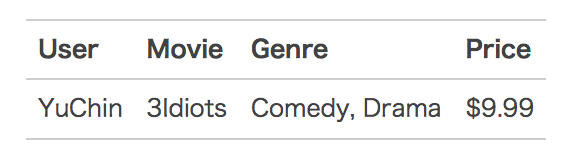• 特征编号：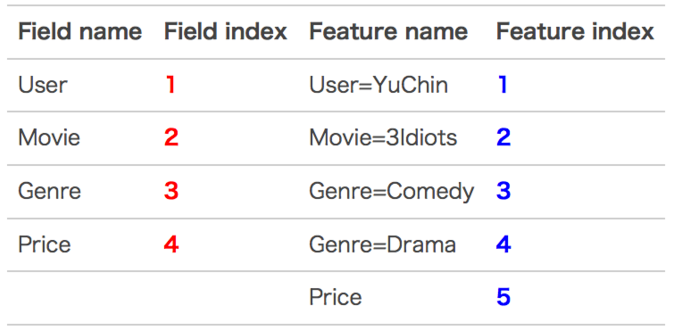• 特征组合：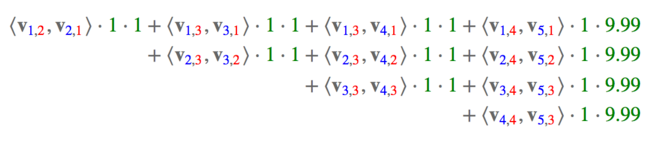• 样本归一化：FFM默认是进行样本数据的归一化，即 为真；若此参数设置为假，很容易造成数据inf溢出，进而引起梯度计算的nan错误。因此，样本层面的数据是推荐进行归一化的。
• 特征归一化：CTR/CVR模型采用了多种类型的源特征，包括数值型和categorical类型等。但是，categorical类编码后的特征取值只有0或1，较大的数值型特征会造成样本归一化后categorical类生成特征的值非常小，没有区分性。例如，一条用户-商品记录，用户为“男”性，商品的销量是5000个（假设其它特征的值为零），那么归一化后特征“sex=male”（性别为男）的值略小于0.0002，而“volume”（销量）的值近似为1。特征“sex=male”在这个样本中的作用几乎可以忽略不计，这是相当不合理的。因此，将源数值型特征的值归一化到 是非常必要的。
• 省略零值特征：从FFM模型的表达式可以看出，零值特征对模型完全没有贡献。包含零值特征的一次项和组合项均为零，对于训练模型参数或者目标值预估是没有作用的。因此，可以省去零值特征，提高FFM模型训练和预测的速度，这也是稀疏样本采用FFM的显著优势。

## 三、DeepFM

FM通过对于每一位特征的隐变量内积来提取特征组合，最后的结果也不错，虽然理论上FM可以对高阶特征组合进行建模，但实际上因为计算复杂度原因，一般都只用到了二阶特征组合。对于告诫特征组合来说，我们很自然想到多层神经网络DNN

### FM的结构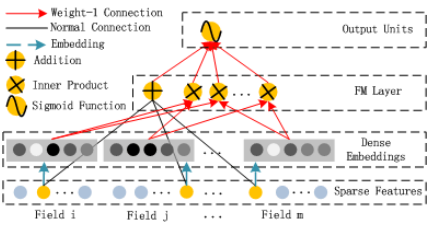### DNN结构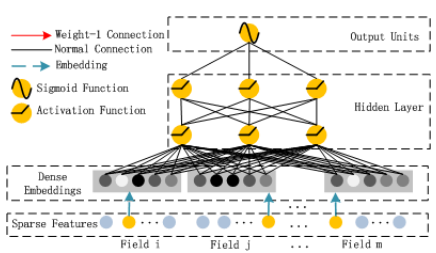### DeepFM结构

FM和DNN的特征结合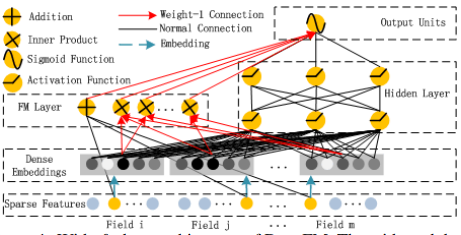DeepFM目的是同时学习低阶和高阶的特征交叉，主要由FM和DNN两部分组成，底部共享同样的输入。模型可以表示为：

$\stackrel{^}{y}=sigmoid\left({y}_{FM}+{y}_{DNN}\right)$

FM部分

${y}_{FM}=+\sum _{i=1}^{d}\sum _{j=i+1}^{d}<{V}_{i},{V}_{j}>{x}_{i}\cdot {x}_{j}$

Deep部分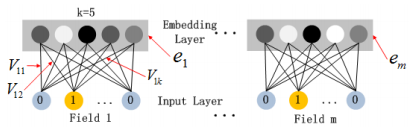1） 尽管不同field的输入长度不同，但是embedding之后向量的长度均为k
2） 在FM中得到的隐变量${V}_{ik}$$V_{ik}$现在作为嵌入层网络的权重

${a}^{\left(l+1\right)}=\sigma \left({W}^{\left(l\right)}{a}^{\left(l\right)}+{b}^{\left(l\right)}\right)$

${y}_{DNN}={W}^{|H|+1}\cdot {a}^{|H|}+{b}^{|H|+1}$

|H|为隐藏层层数

### 模型对比